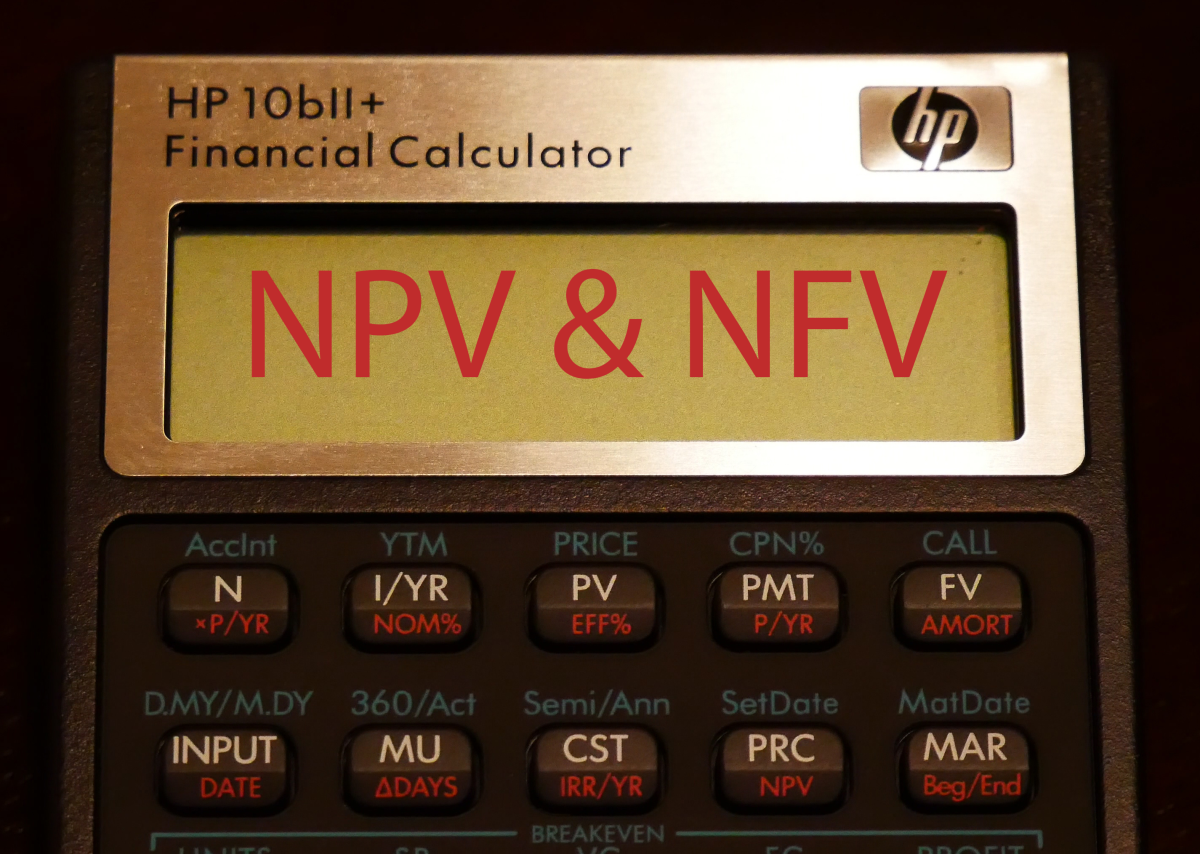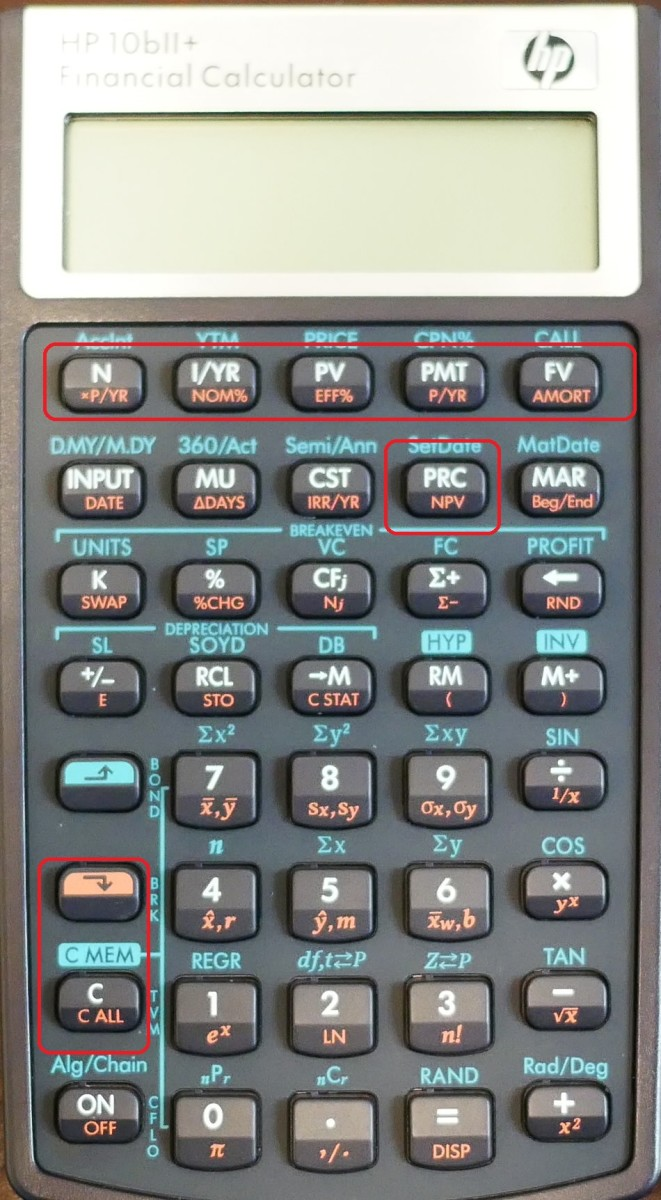Updated:
Original:

# Using an HP 10bII Calculator to Find NPV and NFV With Uneven Cash Flows

Joshua is a graduate student at the USF. He has interests in business technology, analytics, finance, and lean six sigma.The above illustration shows s HP 10bII calculator. There is no easier way to calculate NPV or NFV than with a calculator.Created by Joshua Crowder

## Calculating Net Present Value and Net Future Value

Without getting into the fancy equations, I'm going to show you how to plug numbers into an HP bII+ calculator to find net present value (NPV) and net future value (NFV). If you are following along with a calculator, make sure the calculator is set for payments to occur at the end of the month. Also, make sure that that periods per year is set to 1.

The analysis of investment projects is carried out using discounted cash flow (DCF) analysis. Performance criteria such as net present value (NPV) need to be known to understand if a project is worth undertaking. NFV may be obtained indirectly by compounding the NPV by the number of years of the project life.

### First, Clear the Calculator

To find the net present value of cash payments, we need to first clear the calculator by pressing the orange shift key, then press the "C ALL" key. Next, follow the instructions to save cash flow and interest data from the example to the calculator.NPV calculation formula as well as the NFV calculation can be made with a calculator. This photo of an HP 10bII+ Calculator show the exact buttons that are used to calculate NFV and NPV.Created by Joshua Crowder

## Example

Imagine that you will receive \$100 at the end of each year for the next 3 years, \$300 at the end of Year 4, \$600 at the end of Year 5, and \$700 at the end of Year 6. At the time, other investments of equal risk earn 12% annually. What is its PV?

• Step 1: Type 0, then press the "CFj" button. Because the payments are being made at the end of the year, we won't have a payment for year zero. All payments will be positive since they are cash inflows.
• Step 2: Type 100, then press "CFj" button three times. The first time you press "CFj", you will save 100 as the first cash flow. The next two times you press "CFj", you will save 100 as cash flow 2 and 3.
• Step 3: Type 300, then press "CFj". 300 is saved as cash flow 4
• Step 4: Type 600, then press "CFj". 600 is saved as cash flow 5
• Step 5: Type 700, then press "CFj". 700 is saved as cash flow 6
• Step 6: Type 12, then press "I/Y".

### Solving for NPV

Now that all of our data is in the calculator, we can solve for NPV. Press the orange shift button, then press the "NVP" button labeled in orange. Your answer should be \$1,125.94.

### Solving for NFV

Now we can solve for NFV. Since this particular calculator does not have an NFV button, we are going to have to utilize the TVM keys to find our answer. Start off by pressing the orange shift key, then press the "C ALL" button to clear the TVM function.

• Step 1: Type 6, then press the "N" button
• Step 2: Type 12, then press the "I/Y" button
• Step 3: Type -1,125.94, then press the "PV" button
• Step 4: Type 0, then press the PMT button

Now you can solve for the FV by pressing the "FV" button. The answer should be \$2,222.41.

This content is accurate and true to the best of the author’s knowledge and is not meant to substitute for formal and individualized advice from a qualified professional.